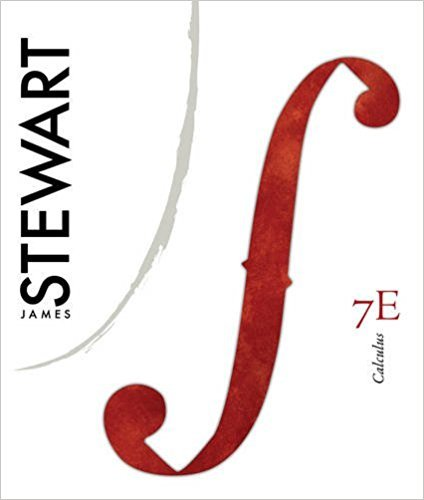×
Get Full Access to Calculus, - 7 Edition - Chapter 2 - Problem 48
Get Full Access to Calculus, - 7 Edition - Chapter 2 - Problem 48

×

# Find an equation of the tangent to the curve at | Ch 2 - 48ISBN: 9780538497817 125

## Solution for problem 48 Chapter 2

Calculus, | 7th Edition

• Textbook Solutions
• 2901 Step-by-step solutions solved by professors and subject experts
• Get 24/7 help from StudySoup virtual teaching assistantsCalculus, | 7th Edition

4 5 1 237 Reviews
19
5
Problem 48

Find an equation of the tangent to the curve at the given point. x 2 1 x 2

Step-by-Step Solution:
Step 1 of 3

Step 2 of 3

Step 3 of 3

##### ISBN: 9780538497817

Unlock Textbook Solution